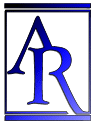Annu. Rev. Astron. Astrophys. 1984. 22: 157-184 Copyright © 1984 by Annual Reviews. All rights reserved

2.5. Imlications

The discussion above makes clear the nature of the available alternatives if one is to avoid the conclusion that the Universe originates in a SHBB. One can question

1. the nature of the observed redshifts (Equations 1-3) by adopting either a different theory of light propagation or a different astrophysical interpretation;

2. the conservation laws and/or gravitational field equations (Equations 5-7);

3. the nature of matter in the Universe, e.g. by assuming some effective contribution to the matter stress tensor that violates the energy conditions (Equation 9).

In each case one can avoid the existence of an initial singularity but must carefully consider if the resulting theory gives a satisfactory account of the microwave background radiation and element abundances. An additional possibility is to consider different geometric assumptions. One can question

4. the assumption of exact spatial homogeneity and isotropy. Then at least one of the shear, vorticity, and acceleration will be nonzero (so Equation 10 does not follow from Equation 8). Singularities will still occur in the past (45, 102), but they can be so different from the SHBB in their geometry and physics as to represent quite different initial situations.